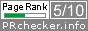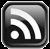# LEARN MS-ACCESS TIPS AND TRICKS

Learn Microsoft Access Advanced Programming Techniques, Tips and Tricks.

### Introduction.

If you have not seen the earlier Posts on Microsoft Access Class Module, please go through them before continuing, the links are given below.

The ClsArea Class can perform as a Base Class in another Class Object, the calculation performed by the base class can be used as part of the new object’s calculations. For example, It can be part of an Object that calculates Volume of something,

The  dbl in dblLength and dblWidth Property Procedure Names for Get/Let Procedures are simply an indication that the Class Object expects the Length and width Values in double precision numbers.  Similarly, If we change the Property Procedure Names to Quantity and UnitPrice then the first value multiplied by the second value gives us the Total Price of some item.

It simply means that you can use the ClsArea Class as a base class, wherever you need the result of first value multiplied by the second value, like Total Price * Tax Rate to calculate tax amount or Total Price * Discount Rate to find Discount Amount and so on.

Even though we have developed a simple Class Module it can be part of many other derived Classes.  The possibilities are open to your imagination and creativity.

Our ClsArea Class calculates area of materials, Rooms or similar items with Length and Width Properties only.  It doesn't calculate area of Triangle or Circle.  But, it can be part of a new Class Object that calculate Volume of Rooms, Warehouses to find storage capacity.  For that we need one more value Height of Room, Warehouse etc.

### The Volume Class: ClsVolume.

Let us create a new Class Module ClsVolume, using the ClsArea as Base Class.  Insert a Class Module and change it’s Name Property to ClsVolume.  Type or Copy and Paste the following Code into the Class Module.

```Option Compare Database
Option Explicit

Private p_Area As ClsArea
Private p_Height As Double

Private Sub Class_Initialize()
Set p_Area = New ClsArea
End Sub

Private Sub Class_Terminate()
Set p_Area = Nothing
End Sub

Public Property Get dblHeight() As Double
dblHeight = p_Height
End Property

Public Property Let dblHeight(ByVal dblNewValue As Double)
Do While Val(Nz(dblNewValue, 0)) <= 0
dblNewValue = InputBox("Negative/0 Values Invalid:", "dblHeight()", 0)
Loop
p_Height = dblNewValue
End Property

Public Function Volume() As Double

If (p_Area.Area() > 0) And (p_Height > 0) Then
Volume = p_Area.Area * p_Height
Else
MsgBox "Enter Valid Values for Length,Width and Height.", , "ClsVolume"
End If

End Function

```

The ClsVolume Class Object’s Code is not yet complete.  Let us examine the Code line-by-line.  In the third line declared as Private Property p_Area of ClsArea Class Object.

Next line, declares a Private Property with the name p_Height of data type Double.

### The Class_Initialize() and Class_Terminate() Sub-Routines.

The Next two Sub-Routines (Initialize() and Terminate()) are very important here.

The Initialize()  runs and instantiate the ClsArea Object in memory,  when we use and instantiate the ClsVolume Class Object in our Standard Module program.

When we execute the Statement Set ClsVolume = Nothing  in the Standard Module program, to clear the ClsVolume Object from memory, the Terminate() Sub-Routine runs and releases the memory space occupied by the ClsArea Object.

The Property Get dblHeight Procedure returns the value from p_Height Property to the calling Program.

The Property Let dblHeight Procedure validates the value passed to the NewValue parameter and assigns it into the private property p_Height.

The Public Function Volume() calculates the Volume, by calling the p_Area.Area() Function and the returned area value is multiplied by p_Height to calculate Volume, with the expression: Volume = p_Area.Area * p_Height.  But, before executing this statement we are performing a validation check to ensure that p_Area.Area() function returns a value greater than zero, indicating that p_Area.dblLength, p_Area.dblWidth Properties have valid values in them and p_Height property value is greater than zero.

Note:  Since, the p_Area Object of Class ClsArea is defined as Private Property of ClsVolume Class we have to make it’s Properties (strDesc, dblLength, dblWidth and Area() function) visible to the outside world for Get/Let Operations and to return Area Value.  That means we have to define Get/Let Property Procedures for strDesc,  dblLength, dblWidth Properties and Area() function of ClsArea Class Object in ClsVolume Class Module too.

### The Let/Get Property Procedures.

Add the following Property Get/Let Procedures and Area() function to the ClsVolume Class Module Code:

```Public Property Get strDesc() As String
strDesc = p_Area.strDesc
End Property

Public Property Let strDesc(ByVal NewValue As String)
p_Area.strDesc = NewValue
End Property

Public Property Get dblLength() As Double
dblLength = p_Area.dblLength
End Property

Public Property Let dblLength(ByVal NewValue As Double)
p_Area.dblLength = NewValue
End Property

Public Property Get dblWidth() As Double
dblWidth = p_Area.dblWidth
End Property

Public Property Let dblWidth(ByVal NewValue As Double)
p_Area.dblWidth = NewValue
End Property

Public Function Area() As Double
Area = p_Area.Area()
End Function

```

Check the strDesc() Property procedures Get/Let lines of Code.  The usage of Procedure name strDesc is simply a matter of choice, if you want to use a different name you are welcome.  But, the original Property Name we have used in the ClsArea Class is strDesc.  Using that original name here reminds us the relationship with the original ClsArea Class Object.

In the next Get dblLength() Property Procedure, the expression to the right of the = sign p_Area.dblLength reads the dblLength value stored in the ClsArea Class Object and returns to the calling program.

The Let Property Procedure assigns the parameter value in NewValue variable to the p_Area.dblLength Property of ClsArea Class Object.  Here, we are not running any validation check on the received value in NewValue parameter variable.  The Validation check will be performed within the ClsArea Class itself, when we assign the value to p_Area.dblLength property.

Similarly the Get/Let Property Procedures are added for the p_Area.dblWidth Property too,

Next, the p_Area.Area() Function is made visible through the ClsVolume Class Objecct to the calling program.

### The ClsVolume Derived Class Module Code.

The completed code of ClsVolume Class Module is given below.

```Option Compare Database
Option Explicit

Private p_Area As ClsArea
Private p_Height As Double

Private Sub Class_Initialize()

‘Open ClsArea Object in Memory with the name p_Area
Set p_Area = New ClsArea

End Sub

Private Sub Class_Terminate()

‘Removes the Object p_Area from Memory
Set p_Area = Nothing ‘
End Sub

Public Property Get dblHeight() As Double
dblHeight = p_Height
End Property

Public Property Let dblHeight(ByVal dblNewValue As Double)

Do While Val(Nz(dblNewValue, 0)) <= 0
dblNewValue = InputBox("Negative/0 Values Invalid:", "dblHeight()", 0)
Loop
p_Height = dblNewValue

End Property

Public Function Volume() As Double

If (p_Area.Area() > 0) And (Me.dblHeight > 0) Then
Volume = p_Area.Area * Me.dblHeight
Else

MsgBox "Enter Valid Values for Length,Width and Height.",vbExclamation , "ClsVolume"
End If

End Function

‘ClsArea Class Property Procedures and Method are exposed here

Public Property Get strDesc() As String
strDesc = p_Area.strDesc
End PropertyPublic Property Let strDesc(ByVal NewValue As String)
p_Area.strDesc = NewValue
End Property

Public Property Get dblLength() As Double
dblLength = p_Area.dblLength
End Property

Public Property Let dblLength(ByVal NewValue As Double)
p_Area.dblLength = NewValue
End Property

Public Property Get dblWidth() As Double
dblWidth = p_Area.dblWidth
End Property

Public Property Let dblWidth(ByVal NewValue As Double)
p_Area.dblWidth = NewValue
End Property

Public Function Area() As Double
Area = p_Area.Area()
End Function
```

I know, what you are thinking by now: like “this is double work, it is nice if we can, some way, skip these steps of repeating ClsArea Property Procedures again in the ClsVolume Class ”.  Or say, we could have added the dblWidth Property in the ClsArea itself and run the Area() and Volume() methods from there itself, right?

The whole point here is that how a Base Class Object can become part of designing another Class Object.

Remember, the whole idea of designing a Reusable Class Module Object is that the main programs, using the Class Object, will be simple and the intricacies built into the Class Object remains hidden.

Yes, we can do it more than one way, with compact code as well.  We will explore them later, but for now let us continue with our original plan.

### The Main Program that Uses the ClsVolume Class.

Let us test our new ClsVolume Class in main Program in Standard Module.  The sample code is given below.

```Public Sub TestVolume()
Dim vol As ClsVolume

Set vol = New ClsVolume

vol.strDesc = "Warehouse"
vol.dblLength = 25
vol.dblWidth = 30
vol.dblHeight = 10

Debug.Print "Description", "Length", "Width", "Height", "Area", "Volume"

With vol
Debug.Print .strDesc, .dblLength, .dblWidth, .dblHeight, .Area(), .Volume()
End With
End Sub
```

You can see how simple the main program is, without the printing lines?

Copy and Paste the code into a Standard Module.  Press Ctrl+G Keys to display the Debug Window, if it is not already in open state. Click somewhere in the middle of the Code and press F5 Key to run the Code.  The sample output on the Debug Window is shown below.

```Description   Length        Width         Height        Area          Volume
Warehouse      25            30            10            750           7500
```

Description Length Width Height Area Volume
Warehouse 25 30 10 750 7500

### Validation Checks Performance Tests.

We will run tests to see that the Base Class ClsArea Class’s input value validation check works when values are passed to it through the ClsVolume Class. We have performed some validation checks in the Area() and Volume() functions too.

Let us try them one by one:

First we will pass a negative value to ClsArea.dblLength property through the ClsVolume Class.  It should trigger the error message and open up the Inputbox() function within the Do While…Loop to input correct value.

1.  Replace the Value 25, in the line Vol.dblLength = 25,  with –5 and press F5 Key to run the Code.

The validation check will trigger the error and will ask for a value greater than Zero.  Enter a value greater than 0.  After that restore the value 25 in the line, replacing –5.

2.  Disable the line Vol.dblHeight = 10 by inserting a comment symbol (‘) at the beginning of the line as shown: ‘Vol.dblHeight = 10.  After the change press F5 Key to run the Code.

Since, there is no input value passed to the Property the Vol.Volume() function will generate an Error saying that all the three Properties: dblLength, dblWidth and dblHeight, should have values in them to run the Volume function.

Similarly, you may check the Vol.Area() Function’s performance too.

We can create a data printing Function and pass the ClsVolume Object as parameter to the function and print the values in the Debug Window.

### The Changed Code of Main Programs.

The changed Code for both Programs are given below:

```Public Sub TestVolume()
Dim Vol As ClsVolume

Set Vol = New ClsVolume

Vol.strDesc = "Warehouse"
Vol.dblLength = 25
Vol.dblWidth = 30
Vol.dblHeight = 10

Call CVolPrint(Vol)

Set Vol = Nothing

End Sub

```

```Public Sub CVolPrint(volm As ClsVolume)

Debug.Print "Description", "Length", "Width", "Height", "Area", "Volume"
With volm
Debug.Print .strDesc, .dblLength, .dblWidth, .dblHeight, .Area, .Volume
End With

End Sub

```

Next week we will build the Volume Class Object with less Code.

### The Links of All Pages on this Topic.

Share:

Comments subject to moderation before publishing.

## TranslateSubscribe in a readerYour email address:

Delivered by FeedBurner

## Featured Post

### Word Mail-Merge with Ms-Access Table

Introduction. Form Letters. Form Letters are prepared from a standard template merged with addressee related information filled in on each l...